# Properties of Matter Properties and Changes Physical Properties

• Slides: 21Properties of Matter Properties and ChangesPhysical Properties n Observation does not alter the identity of the fundamental properties n n n color phase mass volume density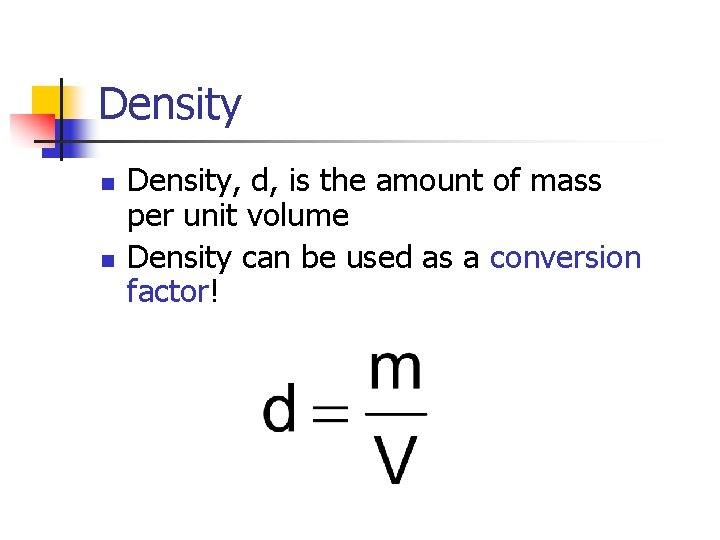Density n n Density, d, is the amount of mass per unit volume Density can be used as a conversion factor!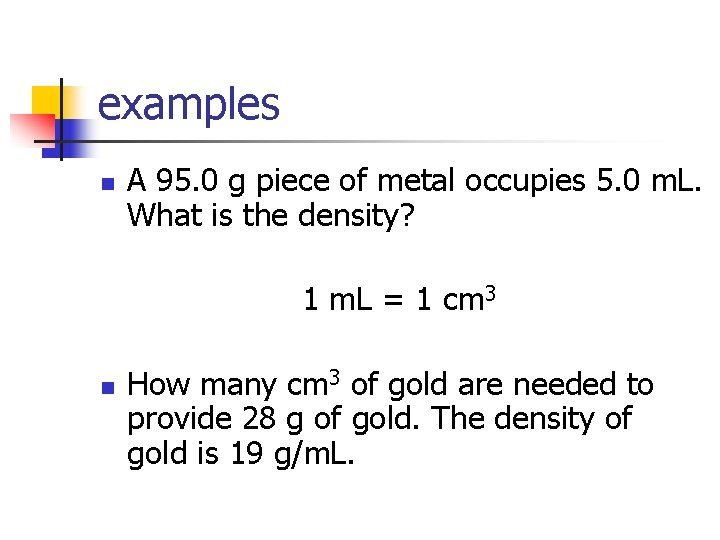examples n A 95. 0 g piece of metal occupies 5. 0 m. L. What is the density? 1 m. L = 1 cm 3 n How many cm 3 of gold are needed to provide 28 g of gold. The density of gold is 19 g/m. L.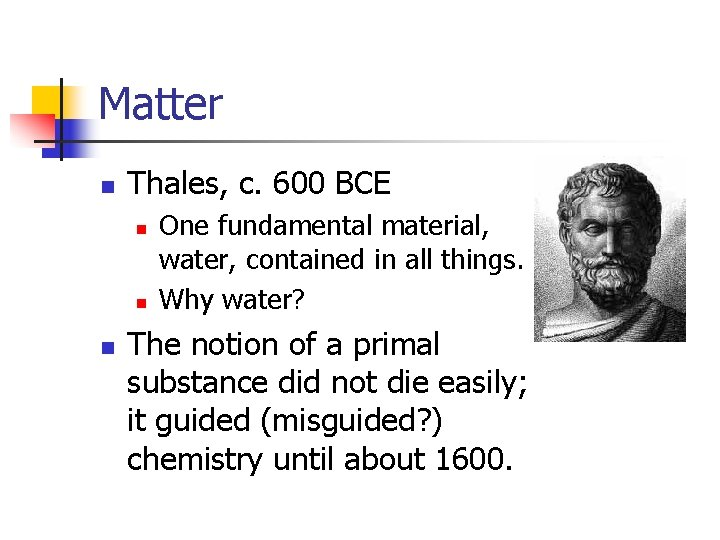Matter n Thales, c. 600 BCE n n n One fundamental material, water, contained in all things. Why water? The notion of a primal substance did not die easily; it guided (misguided? ) chemistry until about 1600.Matter n n Democritus & Leucippus – Atoms!! n All matter is composed of solid atoms and empty space. The atoms’ shapes, and how they connect determine the properties of the substance. The idea was not well-received. Aristotle argued that air would rush in to fill any empty space.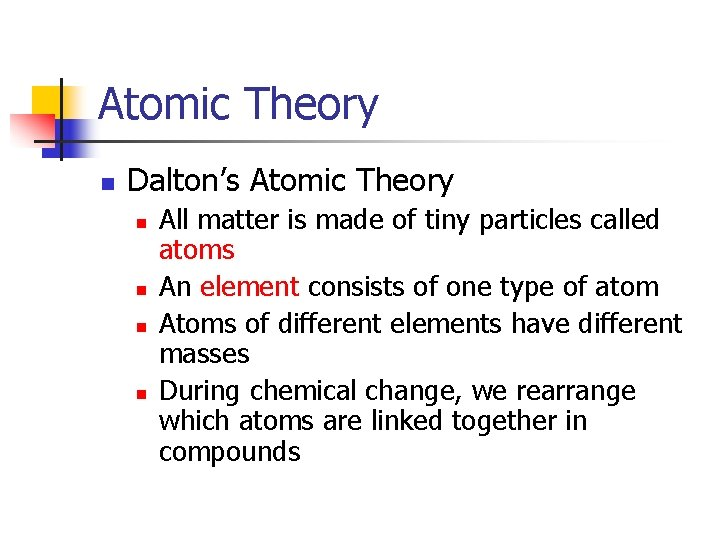Atomic Theory n Dalton’s Atomic Theory n n All matter is made of tiny particles called atoms An element consists of one type of atom Atoms of different elements have different masses During chemical change, we rearrange which atoms are linked together in compoundsAtomic Structure n Atoms contain three particles n n n protons – positive charge and mass of about 1 amu neutrons – no charge and mass of about 1 amu electrons – negative charge and mass about 1800 times less than 1 amu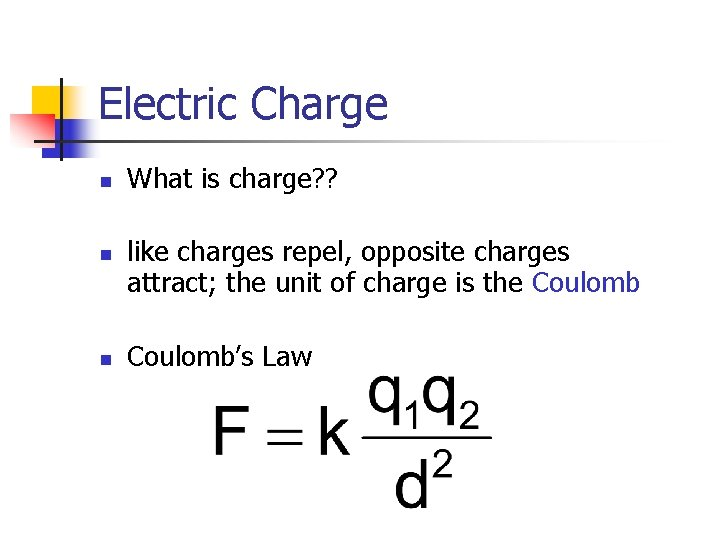Electric Charge n n n What is charge? ? like charges repel, opposite charges attract; the unit of charge is the Coulomb’s Law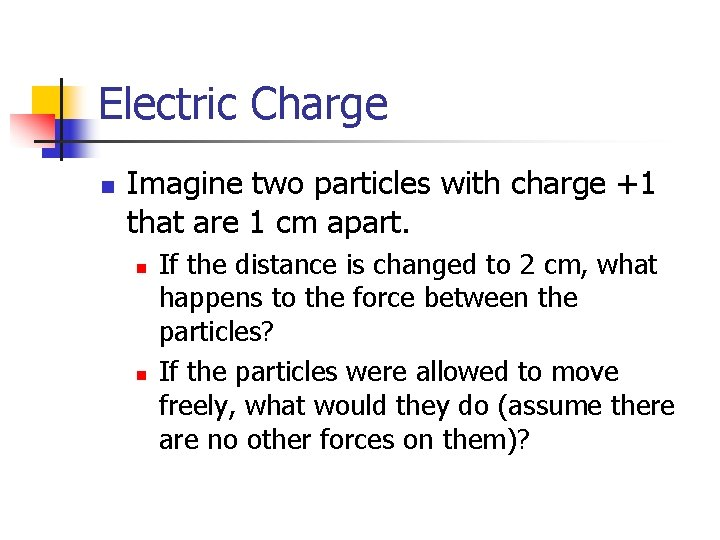Electric Charge n Imagine two particles with charge +1 that are 1 cm apart. n n If the distance is changed to 2 cm, what happens to the force between the particles? If the particles were allowed to move freely, what would they do (assume there are no other forces on them)?Atomic Structure n n n The protons and neutrons occupy a tiny region at the center of the atom called the nucleus. The electrons occupy a huge region around the nucleus called the electron cloud. Electrons DO NOT orbit the nucleus the way planets orbit the sun!!Electric Charge n Why does a negatively charged balloon stick to a wall? n Is it because the wall is positively charged? n NO!! n n The wall, however, is made of atoms and atoms contain charged particles The atoms of the wall are polarized by the balloon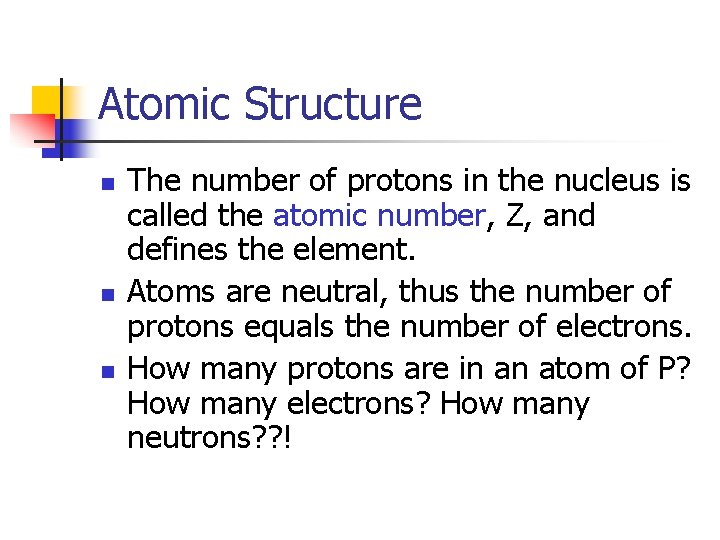Atomic Structure n n n The number of protons in the nucleus is called the atomic number, Z, and defines the element. Atoms are neutral, thus the number of protons equals the number of electrons. How many protons are in an atom of P? How many electrons? How many neutrons? ? !Isotopes n n If two atoms contain the same number of protons, they are atoms of the same element. If they have different numbers of neutrons, they are isotopes of each other. Isotopes – atoms of an element that have different masses because they have different numbers of neutrons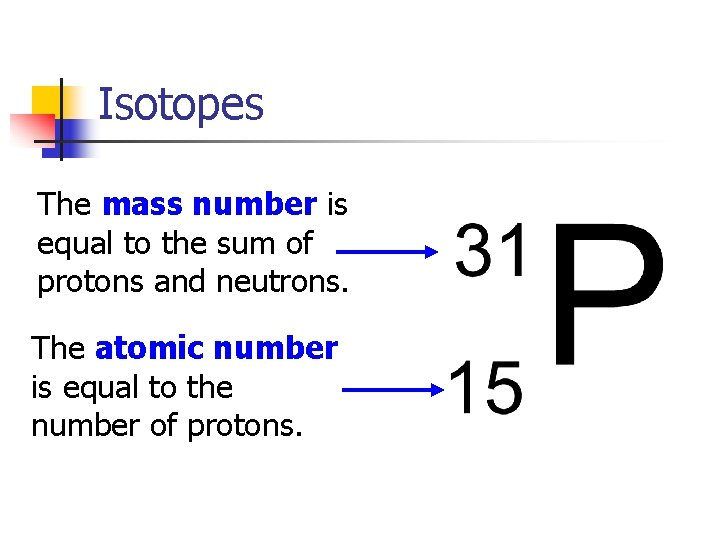Isotopes The mass number is equal to the sum of protons and neutrons. The atomic number is equal to the number of protons.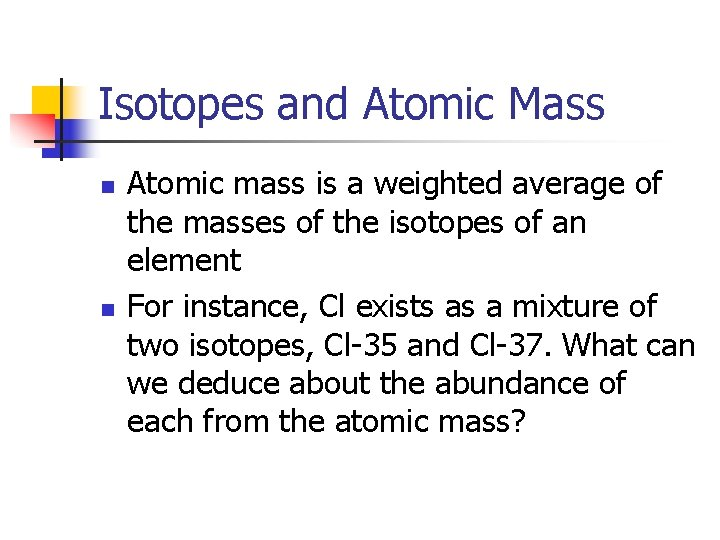Isotopes and Atomic Mass n n Atomic mass is a weighted average of the masses of the isotopes of an element For instance, Cl exists as a mixture of two isotopes, Cl-35 and Cl-37. What can we deduce about the abundance of each from the atomic mass?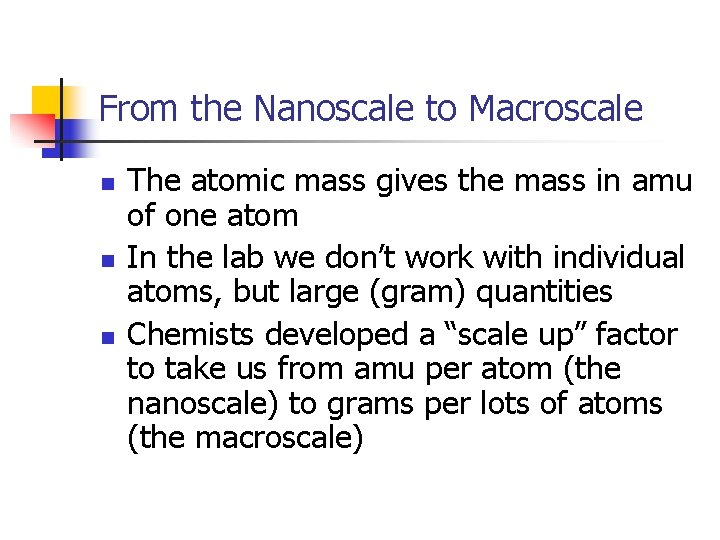From the Nanoscale to Macroscale n n n The atomic mass gives the mass in amu of one atom In the lab we don’t work with individual atoms, but large (gram) quantities Chemists developed a “scale up” factor to take us from amu per atom (the nanoscale) to grams per lots of atoms (the macroscale)The Mole n The mole, mol, is like the “chemists’ dozen” 1 mol = 6. 02 × 1023 Avogadro’s Number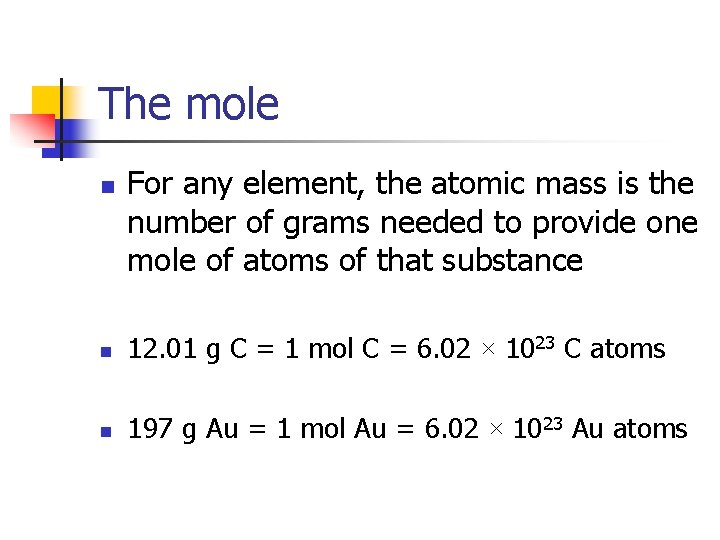The mole n For any element, the atomic mass is the number of grams needed to provide one mole of atoms of that substance n 12. 01 g C = 1 mol C = 6. 02 × 1023 C atoms n 197 g Au = 1 mol Au = 6. 02 × 1023 Au atomsThe mole n n The mole (and Avogadro’s number) gives us a connection between our macroscopic observations and the nanoscale. For any element, given a mass in grams, we can calculate the number of atoms present.examples n If I have 20 g of Al. . . n How many moles of Al is this? n How many Al atoms are present?